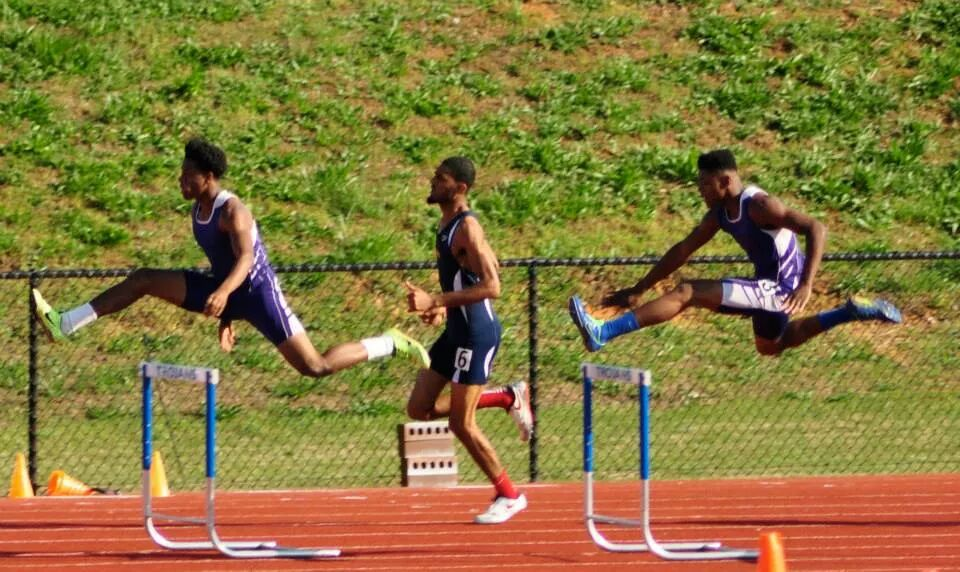# 2A Boys Team Power Rankings Thru 4-2

###Fitzgerald has a dynamic Hurdles duo including Trey Graham and Daniel Rivera

### 2015 ga HS M Outdoor Track Team Scores

Calculated as of April 03, 2015

These rankings were calculated by scoring the rankings as a meet. We scored 10 deep for individuals and 10 deep for relays. For example, for individuals 1st place received 10 points for their team, on down to 1 point for 10th place.

1.Fitzgerald109.5
Chandler Eckler (PV 8 = 8)
Daniel Rivera (300H 8 + TJ 8.5 = 16.5)
Darrius Hampton (HJ 9 = 9)
Gerald Morgan (110H 5 = 5)
James Graham (100m 5 + 200m 2 = 7)
Jd King (LJ 9 = 9)
Kelton Williams (HJ 10 = 10)
Relay Team (15)
Trey Graham (110H 10 + 300H 10 + TJ 10 = 30)

2.Wesleyan School79
Austin Leath (800m 6 = 6)
Bailey Renfroe (1600m 3 + 3200m 5 = 8)
Cedric Lynch (200m 7 + LJ 3 = 10)
Christopher Lee (PV 2.5 = 2.5)
Clay Patrick (300H 5 = 5)
Drew Jones (LJ 6 + TJ 6 = 12)
Drujames Douglas (300H 1 = 1)
Jalen Hodges (HJ 5 = 5)
Jason McClesky (110H 3 = 3)
Jordan Mack (200m 3 = 3)
Kyle Rappe (S 6 = 6)
Nathan Settlage (PV 5.5 = 5.5)
Relay Team (12)
Chris Howard (S 4 + TJ 7 = 11)
Dante Tate (200m 4 + 400m 6 = 10)
Durante Tate (200m 5 = 5)
Imoter Mngerem (D 5 = 5)
Malik Faulk (110H 8 + 300H 3 = 11)
Relay Team (12)
Tucker Pearson (110H 6 + 300H 7 = 13)
4.Bleckley County High School68.5
Anuj Patel (800m 5 = 5)
Austin Cook (3200m 3 = 3)
Carl Ellis Corley (1600m 6 + 3200m 6 = 12)
Clay Blanton (800m 4 = 4)
Cord Yearty (D 7 = 7)
Dayton Cannon (3200m 1 = 1)
Porter Arnold (PV 5.5 = 5.5)
Relay Team (4)
Shamar Slappy (D 10 = 10)
Tae Holiday (LJ 2 = 2)
Tijea Randall (S 7 = 7)
Will Simmons (PV 8 = 8)

 5. Greater Atlanta Christian 66 Darius Slayton (100m 10 + 200m 10= 20) Micah Abernathy (400m 10 = 10) Miller Kettle (1600m 10 + 3200m 9 + 800m 9 = 19) Relay Team (17)
6.Holy Innocents' Episcopal School56.25
Alex Thomas (PV 0.25 = 0.25)
Andrew Coggins (100m 4 + 400m 7 = 11)
Ben Davies (800m 10 + PV 10 = 20)
Braxton Lindley (400m 8 + 800m 8 = 16)
Relay Team (9)

7.Lovett37.75
Alec White (100m 1 = 1)
Charlie Powers (1600m 5 = 5)
Evan Mercer (PV 2.5 = 2.5)
Everett Smulders (1600m 9 + 3200m 4 + 800m 7 = 20)
Harrison Barnes (PV 0.25 = 0.25)
Nicholas Klavohn (1600m 7 + 800m 2 = 9)
8.Thomasville High School37
Andrew Jackson (300H 6 + 400m 5 = 11)
Bryan Kato (D 9 + S 9 = 18)
James Davis (HJ 5 = 5)
Keldren Reddick (800m 3 = 3)
9.Lamar County High School36.5
Akeem Colquitt (D 3 = 3)
Lj Smith (110H 1 + HJ 5 = 6)
Noah Eure (400m 1 + TJ 8.5 = 9.5)
Relay Team (8)
Shon Taylor (300H 2 + LJ 4 = 6)
Tyran Buckner (TJ 4 = 4)
10.East Laurens27
Jaquivus Cannon (100m 9 + 200m 8 = 17)
Relay Team (10)
De'Ontia Butler (S 2 = 2)
Relay Team (6)
Tarik Prince (110H 9 + 300H 9 = 18)
12.Groves25.17
Relay Team (11)
Shabari Davis (200m 6 + HJ 8 = 14)
Vincent Mordecai (HJ 0.17 = 0.17)
13.Bacon County HS23
Drell Greene (HJ 5 + LJ 7 + TJ 5 = 17)
Katron Folsom (HJ 5 = 5)
Trae Stewart (TJ 1 = 1)
Alec Rogers (1600m 2 = 2)
Jack Douglass (3200m 7 = 7)
Max Irvine (1600m 4 = 4)
Michael Easley (LJ 5 = 5)
Xori Johnson (400m 4 = 4)
15.Darlington School18.17
Elijah McKoy (HJ 0.17 = 0.17)
Spencer Fields (1600m 8 + 3200m 10 = 18)
16.Bremen High School16.17
Christain Ray (HJ 0.17 = 0.17)
Kaleb Moten (D 6 + S 10 = 16)
17.Vidalia14.17
Jaquan Cornish (HJ 0.17 = 0.17)
Nathan McBride (200m 9 = 9)
Shon Jones (TJ 3 = 3)
Tyler Campbell (3200m 2 = 2)
17.Jeff Davis14.17
Donald Bonner (400m 2 = 2)
Jarrod Sims (HJ 0.17 = 0.17)
Kolby Carter (110H 4 + 300H 4 = 8)
Relay Team (4)
19.Berrien County HS14
Hunter Tucker (1600m 1 + 3200m 8 = 9)
Keyshawn Prince (S 5 = 5)
20.Chattahoochee County11
Isaiah Captian (D 8 + S 3 = 11)
21.Social Circle High School10
Maurice Lewis (LJ 10 = 10)
22.Washington-Wilkes High School9
Devarious Smith (100m 3 = 3)
Dj Binns (100m 2 = 2)
Jacorey Burley (HJ 2 = 2)
Relay Team (2)
23.Gordon Lee High School8.25
Andrew Towns (PV 8 = 8)
Dillon Williams (PV 0.25 = 0.25)
24.Temple High School8
Hakim Montgomery (100m 7 + 200m 1 = 8)
Marquis Grier (100m 8 = 8)
24.Monticello8
Jay Johnson (LJ 8 = 8)
27.Bryan County HS7.5
Marquez Bostick (S 0.5 = 0.5)
Quran George (110H 7 = 7)
28.Rabun County5.5
Dalton Keener (PV 4 = 4)
Tucker Williams (D 1 + S 0.5 = 1.5)